# Qlik Sense: Sorting by Expression

This can be a very frustrating problem for many Qlik users.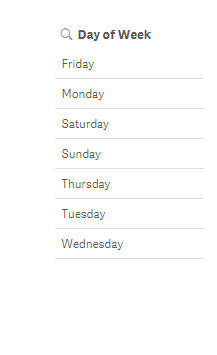I have a list of the days of the week, but as you can see they are not in order. Unfortunately, sorting them alphabetically will not help your situation either. The solution is to Sort by Expression

Go into to edit mode and click on the object in question (in this case a Filter). Now click on Sorting in the right side bar and unclick Auto Sorting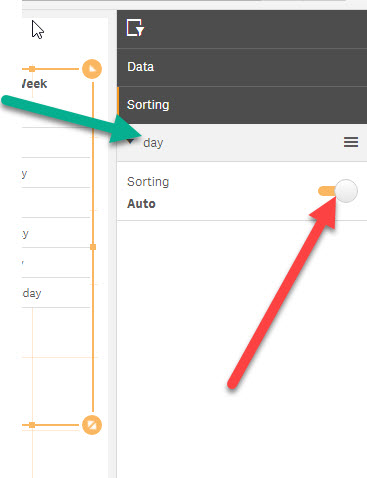Uncheck Sort numerically and Sort alphabetically, and check Sort by expressionClick on the Expression box and the formula window opens.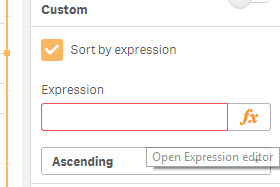The formula we are going to use is the Match() formula:

Syntax: match(field, condition 1, condition 2, etc)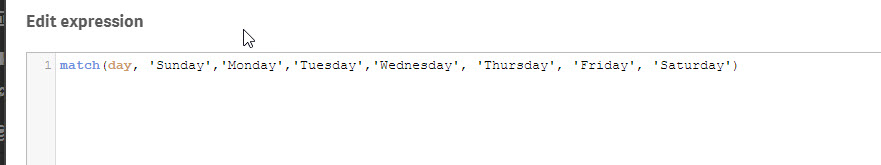Hit apply and check your Filter box.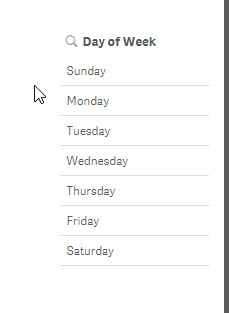# QLIK Sense: If Then Conditional Logic

One place Qlik really shines in my opinion is in their data modeling. While Qlik Sense brought user friendliness to the mix by allowing automatic data model creation, it still allows almost unlimited customization to those looking to peek under the hood.

In today’s example, I am going show you how to utilize a simple IF THEN statement in the data model to make building your visualizations much easier.

This data set is made up data modeled off of cardiac patient alarms. Let’s look at our data:

As you can see, we have 5 columns. Count is just a distinct number for each row, day = day of the week, alarm trim down1 = the alarm condition, Alarm Class = severity of alarm – Red is the most severe with INOP being the least, timeday = time of day the event occurred.The task at hand here is simple enough. We want to analyze alarms on the weekend versus weekday.

Loading the data in Qlik is easy enough. If you have any questions on how to do it, refer to my earlier tutorial on building a Dashboard: QLIK: Build a Dashboard Part 1

Go to the App Overview. Select your new sheet, hit Edit and grab a Bar Chart from the left utility bar. We are going to set the Dimension to Alarm Class and the Measure to Count of alarm trim down1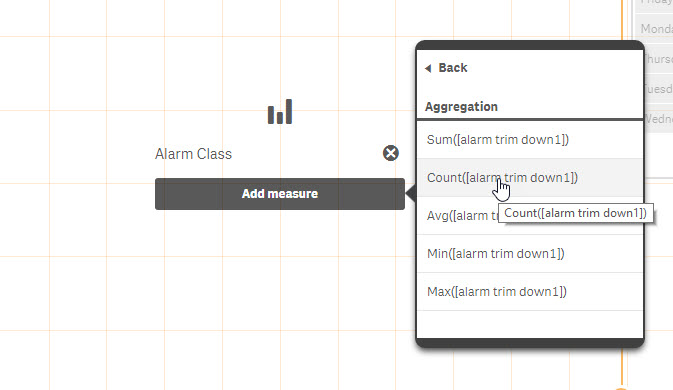This will give you the following Bar Chart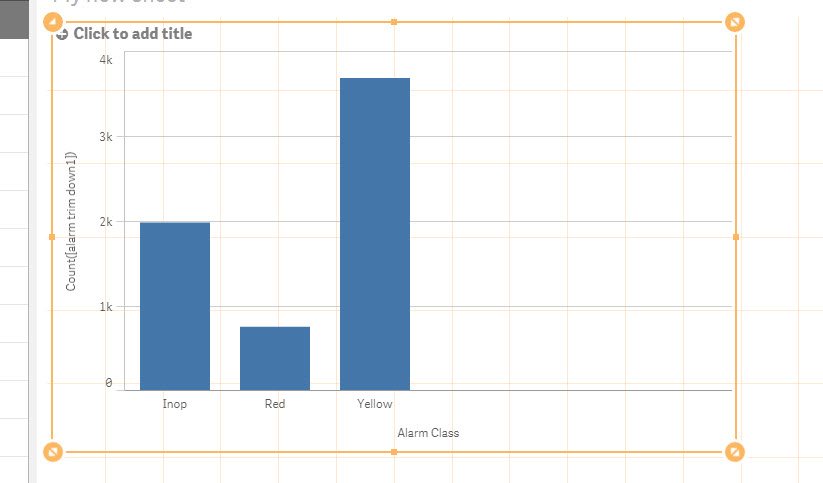Now grab a Filter Pane and drag it onto the sheet. Set its dimension to day.

Now by selecting days from the filter pane you can effectively compare weekdays to weekends. However, it involves a lot of unnecessary clicking for the end user. Let’s try a better method.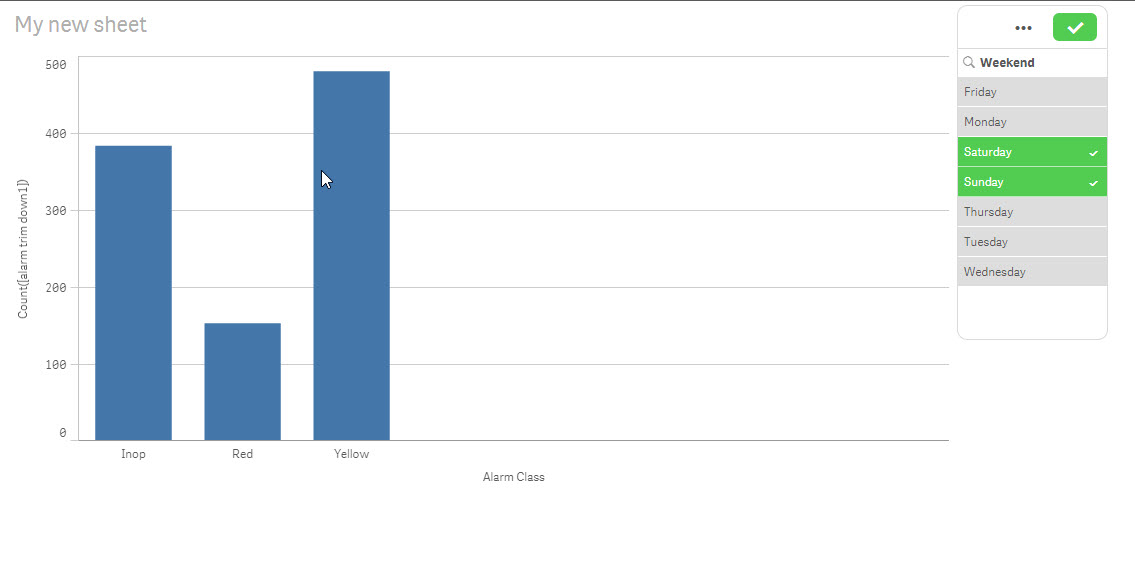Let’s go to the Data load editor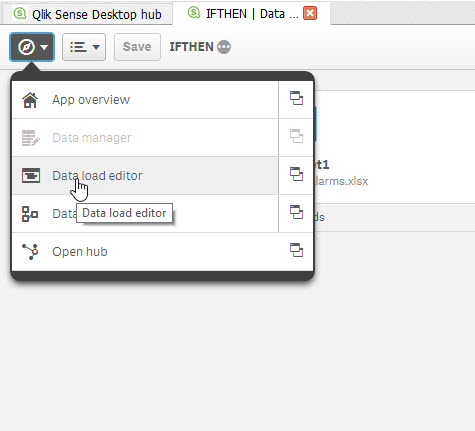click on the Auto-generated section in the left pane.Next click on the Unlock box in the upper right corner. You will be met with a warning window. Just click Okay.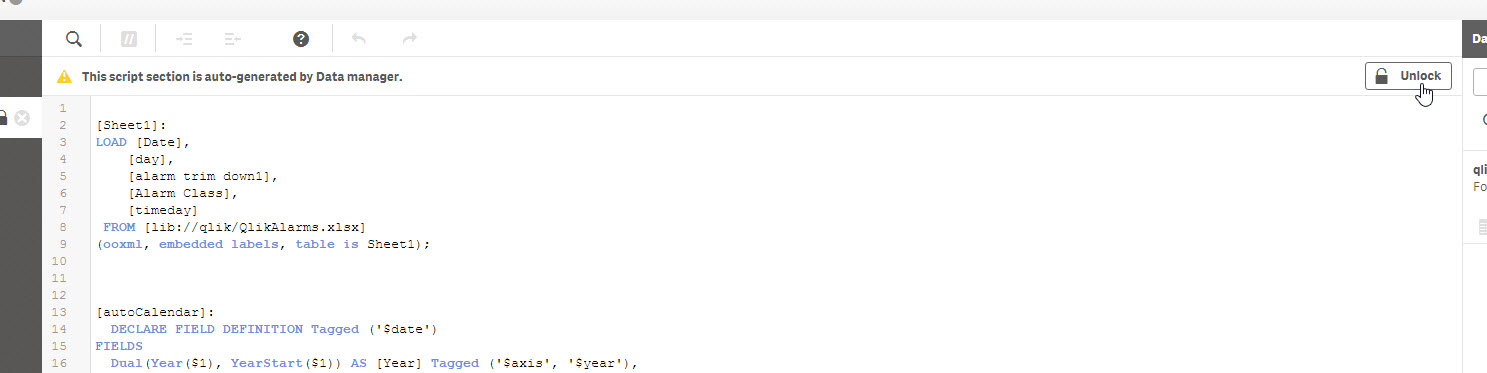Let’s take a look at the Load script. This script was auto-generated by Qlik when you uploaded the Excel file. Note that it looks similar to an SQL script. We are going to LOAD the columns listed below FROM the Excel workbook.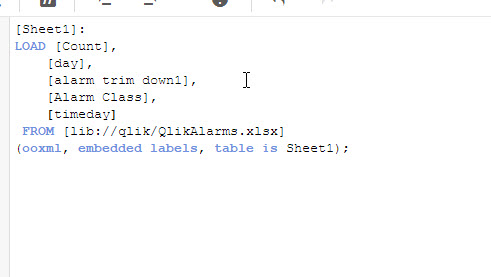What we are going to do next is add a new line to the loading script. This line will be an IF THEN statement.

The syntax is as a follows: if ( conditional statement, THEN, ELSE) as [NAME FOR NEW COLUMN]

In our example I am stating if day is equal to ‘Saturday’ or ‘Sunday’ then 1 else 0 and I am naming this new column Weekend

*** note the , at the end of [timeday]. Make sure you add that there. Qlik will throw an error if the correct syntax is not used.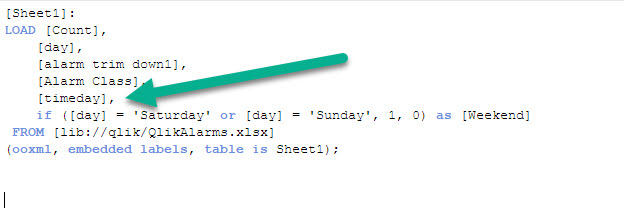Now select Load data. If successful, go back to your App Overview > edit sheet. If not successful, check your syntax!!Let’s replace the day filter pane with a Weekend filter pane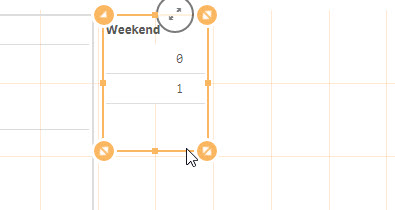Now you can compare weekdays to weekends with just a single click. 1 for weekends and 0 for weekdays.

This still is not ideal. The goal of a good BI solution is usability. The end user should be able to dig into their data without having to spend too much time trying to decipher what built.

Let’s correct this. Go back to data load editor. Let’s change our IF THEN statement to read if day = Saturday or Sunday, then ‘Weekend’, else ‘Weekday’Click Load data and go back to your sheet. Notice the filter pane now shows Weekday and Weekend as your options.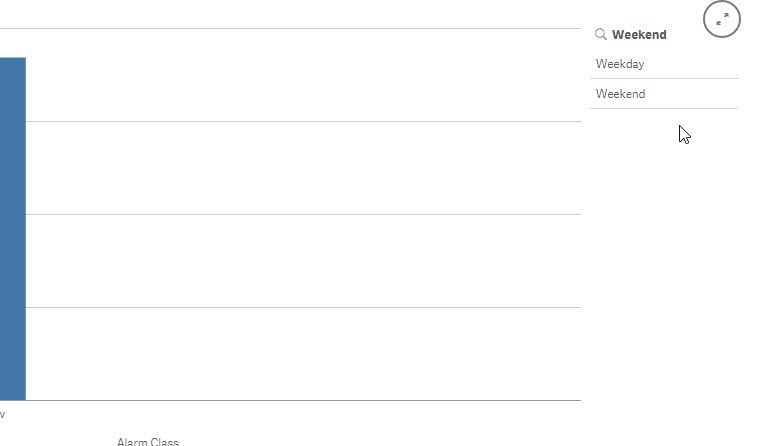# R: Text Mining (Pre-processing)

This is part 2 of my Text Mining Lesson series. If you haven’t already, please check out part 1 that covers Term Document Matrix: R: Text Mining (Term Document Matrix)

Okay, now I promise to get to the fun stuff soon enough here, but I feel that in most tutorials I have seen online, the pre-processing of text is often glanced over. It was always (and often still is) a real sore spot for me when assumptions are made as to my knowledge level. If you are going to throw up a block of code, at least give a line or two explanation as to what the code is there for, don’t just assume I know.

I remember working my way through many tutorials where I was able to complete the task by simply copying the code, but I didn’t have a full grasp of what was happening in the middle. I this lesson, I am going to cover some of the more common text pre-processing steps used in the TM library. I am going to go into some level of detail and make some purposeful mistakes so hopefully when you are done here you will have a firm grasp on this very important step in the text mining process.

Let’s start by getting our libraries

```install.packages("tm") # if not already installed
install.packages("SnowballC")

library(tm)
library(SnowballC)```

Now, let’s load our data. For this lesson we are going to use a simple vector.

```wordVC <- c("I like dogs!", "A cat chased the dog.", "The dog ate a bone.",
"Cats make fun pets.", "The cat did what? Don't tell the dog.",
"A cat runs when it has too, but most dogs love running!")```

Now let’s put this data into a corpus for text processing.

```corpus <- (VectorSource(wordVC))
corpus <- Corpus(corpus)
summary(corpus)```

Here is the outputNow for a little frustration. Let’s say you want to see what is in text document 4. You could try

`inspect(corpus)`

But this will be your output, not really what you are looking for.If you want to see the actual text- try this instead

`corpus[]\$content`

Now you can see the textAs we go through the text pre-processing, we are going to use the following For Loop to examine our corpus

`for (i in 1:6) print (corpus[[i]]\$content)`

Output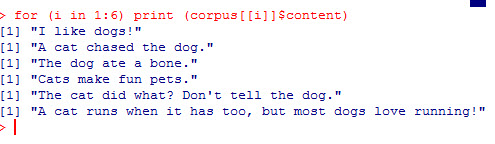### Punctuation

Punctuation generally adds no value to text mining when utilizing standard numeric based data mining algorithms like clustering or classification. So it behooves us to just remove it.

To do so, the tm package has a cool function called tm_map() that we can pass arguments to, such as removePunctuation

``````corpus <- tm_map(corpus, content_transformer(removePunctuation))

```for (i in 1:6) print (corpus[[i]]\$content)```

Note, you do not need the for loop, I am simply running it each time to show you the progress.

Notice all the punctuation is gone now.### Stopwords

Next we are going to get rid of what are known as stopwords. Stopwords are common words such as (the, an, and, him, her). These words are so commonly used that they provide little insight as to the actual meaning of the given text.  To get rid of them, we use the following code.

```corpus <- tm_map(corpus, content_transformer(removeWords),
stopwords("english"))
for (i in 1:6) print (corpus[[i]]\$content)```

If you look at line 2, “A cat chase  dog”, you will the word “the” has been removed. However, if you look at the next line down, you will notice “The” is still there.WHY?

Well it comes down to the fact that computers do not treat T and t as the same letter, even though they are. Capitalized letters are viewed by computers as separate entities. So “The” doesn’t match “the” found in the list of stopwords to remove.

For a full list of R stopwords, go to: https://github.com/arc12/Text-Mining-Weak-Signals/wiki/Standard-set-of-english-stopwords

So how do we fix this?

### tolower

Using tm_map with the “tolower” argument will make all the letters lowercase. If we then re-run our stopwords command, you will see all the “the” are gone

```corpus <- tm_map(corpus, content_transformer(tolower))
corpus <- tm_map(corpus, content_transformer(removeWords),
stopwords("english"))
for (i in 1:6) print (corpus[[i]]\$content)```

Output### stemming

Next we will stem our words. I covered this in the last lesson, but it bears repeating. What stemming does is attempt to remove variants of words. In our example, pay attention to the following words (dog, dogs, cat, cats, runs, running)

```corpus <- tm_map(corpus, stemDocument)
for (i in 1:6) print (corpus[[i]]\$content)```

Notice the words are now (dog, cat, run)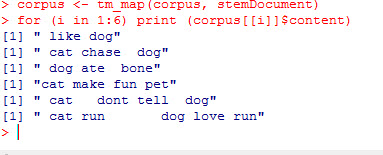### Whitespace

Finally, let’s get rid of all this extra white space we have now.

``````corpus <- tm_map(corpus, stripWhitespace)
for (i in 1:6) print (corpus[[i]]\$content)``````

Output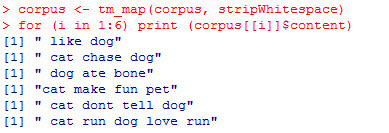### removeNumbers

I didn’t use this argument with my tm_map() function today because I did not have any numbers in my text. But if I, the command would be as follows

`corpus <- tm_map(corpus, content_transformer(removeNumbers))`

# R: Text Mining (Term Document Matrix)

There are a bounty of well known machine learning algorithms, both supervised (Decision Tree, K Nearest Neighbor, Logistical Regression) and unsupervised (clustering, anomaly detection). The only catch is that these algorithms are designed to work with numbers, not text. The act of using numeric based data mining methods on text is known as duo-mining.

So before you can utilize these algorithms, you first have to  transform text into a format suitable for use in these number based algorithms. One of the most popular methods people first learn is how to create a Term Document Matrix (TDM). The easiest way to understand this concept is, of course, to do an example.

```install.packages("tm") # if not already installed
library(tm)```

Now let’s create a simple vector of strings.

```wordVC <- c("I like dogs", "A cat chased the dog", "The dog ate a bone",
"Cats make fun pets")```

Now we are going to place the strings into a data type designed for text mining (from the tm package) called corpus. A corpus simply means the full collection of text you want to work with.

```corpus <- (VectorSource(wordVC))
corpus <- Corpus(corpus)
summary(corpus)```

Output:

``` Length Class Mode
1 2 PlainTextDocument list
2 2 PlainTextDocument list
3 2 PlainTextDocument list
4 2 PlainTextDocument list```

As you can see from the summary, the corpus classified each string in the vector as a PlaintextDocument.

Now let’s create our first Term Document Matrix

```TDM <- TermDocumentMatrix(corpus)
inspect(TDM)```

Output: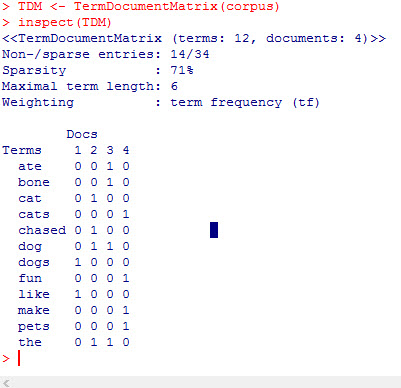As you can see, we now have a numeric representation of our text. Each row represents a word in our text and each column represents an individual sentence. So if you look at the word dog, you can see it appears once in sentence 2 and 3, (0110). While bone appears once in sentence 3 only (0010).

## Stemming

One immediate issue that jumps out at me is that R now sees the words cat & cats and dog & dogs as different words, when really cats and dogs are just the plural versions of cat and dog. Now there may be some more advanced applications of text mining that you would want to keep the two words separate, but in most basic text mining applications, you would want to only keep one version of the word.

Luckily for us, R makes that simple. Use the function tm_map with the argument stemDocument

`corpus2 <- tm_map(corpus, stemDocument)`

make a new TDM

```TDM2 <- TermDocumentMatrix(corpus2)
inspect(TDM2)```

Now you see only the singular of cat and dog exist in our list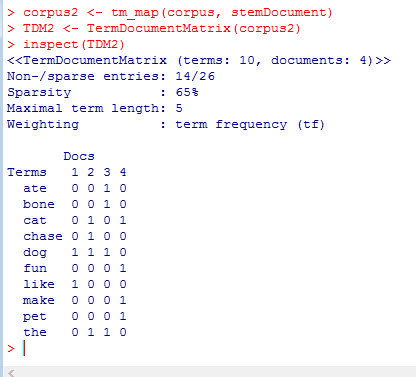If you would like, you can also work with the transpose of the TDM called the Document Term Matrix.

```dtm = t(TDM2)
inspect(dtm)```

dfsaI’ll get deeper into more pre-processing tasks, as well as ways to work with your TDM in future lessons. But for now, practice making TDMs see if you can think of ways that you can use TDMs and DTMs with some machine learning algorithms you might already know (decision trees, logistic regression).

# Excel: Copy, Cut, Paste, and Format Painter

This is a very basic introduction into Excel. I am going to start with the upper left hand corner of the Ribbon bar: the Clipboard region.While I am aware most of you already know how to use these features, my website is for everyone, including the most base beginner. I remember the frustration of learning the fundamentals of analytics from websites that assumed I already had a PhD and 10 years of work experience in the area.

So feel free to skip this, or take a few minutes to read through, you might be surprised. There may be some tricks in this little corner of Excel you were not aware of.

## Cut, Copy, and Past

These 3 features are ambiguous with computer use dating back to days DOS. As a matter of fact, the old keyboard shortcuts used back then still work.

Cut (Ctrl-X) – deletes the highlighted text and stores it in local memory (a clipboard)

Copy (Ctrl-C) – leaves highlighted text as is, but saves a copy of it into local memory

Paste(Ctrl-V) – pastes the contents of the clipboard into the spot you have chosen.

Below are two examples

### CUT

Start by highlight the rows A:1 -A:4, click Cut (or Ctrl-X). Now Select Cell C1. Click Paste (or Ctrl-V). Notice column A is now empty.

### COPY

Start by highlight the rows A:1 -A:4, click Copy (or Ctrl-C). Now Select Cell C1. Click Paste (or Ctrl-V). Notice Column C is now a Copy of Column A

## Clipboard

If you click on the bottom right of the Clipboard box, the clipboard window opens up, showing your the current contents saved to the clipboard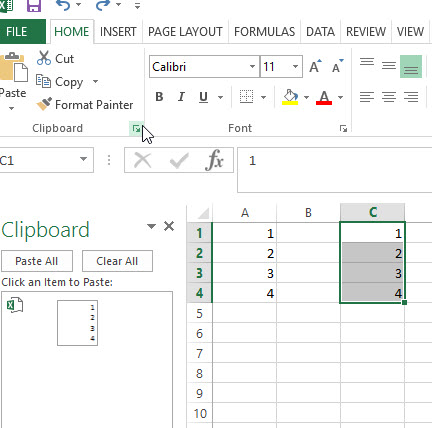So now if I add some letters to column B and copy it, that will end up in the Clipboard as well. Notice the original data is still in the clipboard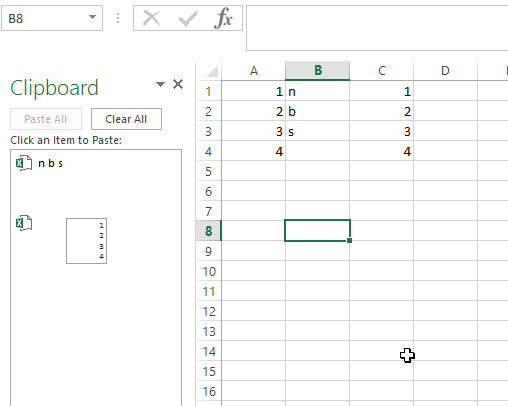Now when you want to paste, you can choose which item in the clipboard to paste. Without using the clipboard, Excel will paste the most recent item added to the clipboard by default.## Copy as Picture

You might notice a drop down arrow next to Copy in the Ribbon Bar. If you click on it, you will see Copy as Picture as an option. This is great when working with Charts. This saves the data as a picture or bitmap so when you paste it elsewhere it will not be affected by changes to the source data.

What I mean by that is, looking at the chart below, column b has a value of 2. If I change that 2 to a 4 in the data table this chart was created from the bar representing b would change to 4 (so would any copied charts). But a chart copied “as picture” would not change. Imagine it to be like a screen shot.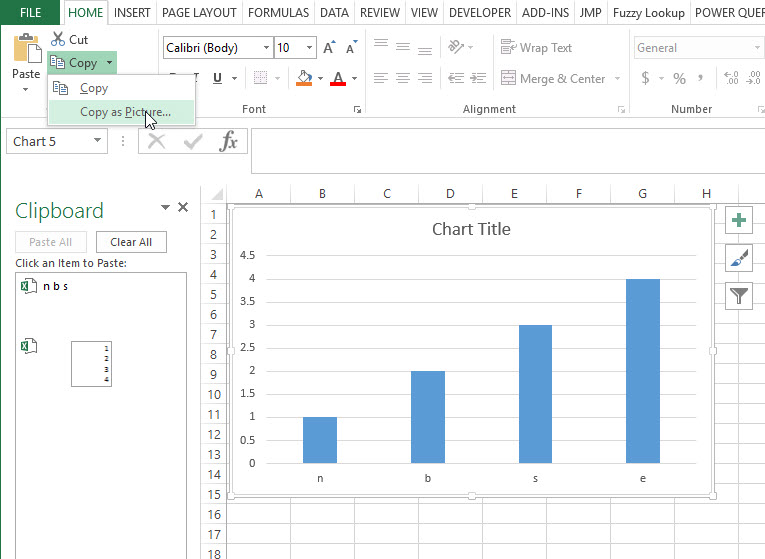## Paste Special

Notice the options below Paste when you hit the drop down arrow. Some of the more popularly used are Transpose (turns a vertical list to horizontal list and vise versa). Another popular option is to paste “values”. This is useful when trying to copy a calculated value where all you want is the number (not the formula who made it).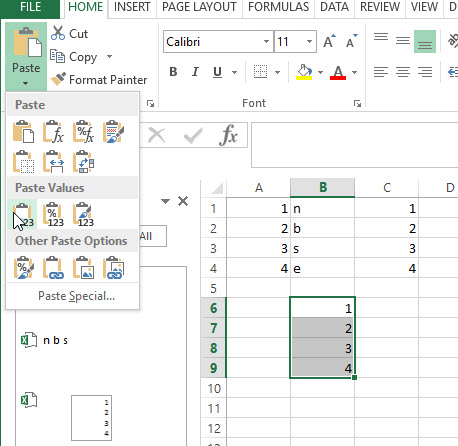## Format Painter

Format Painter can help you repeat text and color formatting with just a few clicks

Start by adding color and font bolding to a set of cells. Highlight those cells and click Format Painter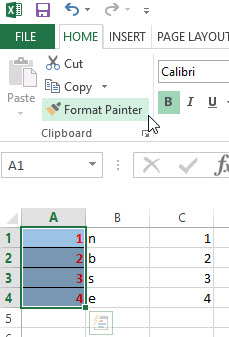You will now see your cursor is paint brush. Find a target you want to duplicate your formatting to and click on it.And now it looks the same.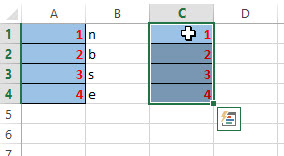# R: Creating a Word Cloud

Word Clouds are great visualization techniques for dealing with text analytics. The idea behind them is they display the most common words in a corpus of text. The more often a word is used, the larger and darker it is.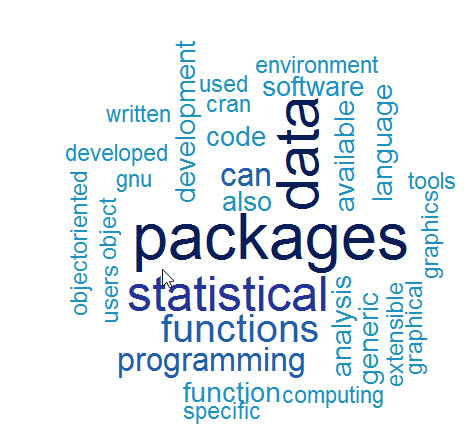Making a word cloud in R is relatively easy. The tm and wordcloud libraries from R’s CRAN repository is used to create one.

```library(tm)
library(wordcloud)

```

If you do not have either of these loaded on your machine, you will have to use the following commands

```install.packages("tm")
install.packages("wordcloud")```

Now in order to make a word cloud, you first need a collection of words. In our example I am going to use a text file I created from the Wikipedia page on R.

Now let’s load the data file.

```text <- readLines("rWiki.txt")
 "R is a programming language and software environment
 "The R language is widely used among statisticians and
 "Polls, surveys of data miners, and studies of scholarly
 "R is a GNU package. The source code for the R
 "General Public License, and pre-compiled binary versions
 "R is an implementation of the S programming language "
>```

Notice each line in the text file is an individual element in the vector –  text

Now we need to move the text into a tm element called a Corpus. First we need to convert the vector text into a VectorSource.

```wc <- VectorSource(text)
wc <- Corpus(wc)```

Now we need to pre-process the data. Let’s start by removing punctuation from the corpus.

`wc <- tm_map(wc, removePunctuation)`

Next we need to set all the letters to lower case. This is because R differentiates upper and lower case letters. So “Program” and “program” would treated as 2 different words. To change that, we set everything to lowercase.

`wc <- tm_map(wc, content_transformer(tolower))`

Next we will remove stopwords. Stopwords are commonly used words that provide no value to the evaluation of the text. Examples of stopwords are: the, a, an, and, if, or, not, with ….

`wc <- tm_map(wc, removeWords, stopwords("english"))`

Finally, let’s strip away the whitespace

`wc <- tm_map(wc, stripWhitespace)`

Now let us make our first word cloud

The syntax is as follows – wordcloud( words = corpus, scale = physical size, max.word = number of words in cloud)

`wordcloud(words = wc, scale=c(4,0.5), max.words=50)`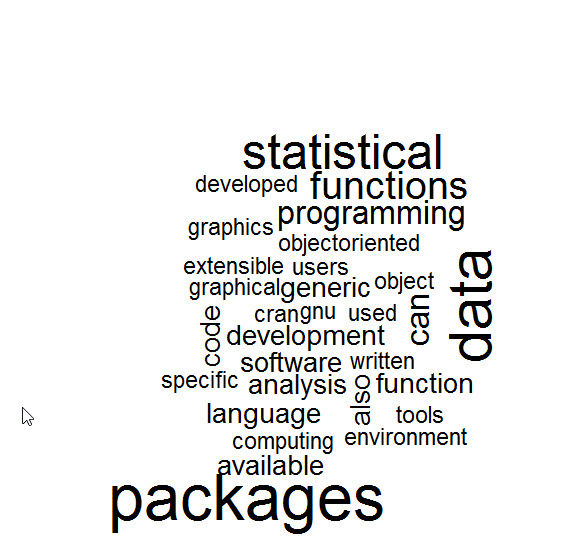Now we have a word cloud, let’s add some more elements to it.

random.order = False brings the most popular words to the center

`wordcloud(words = wc, scale=c(4,0.5), max.words=50,random.order=FALSE)`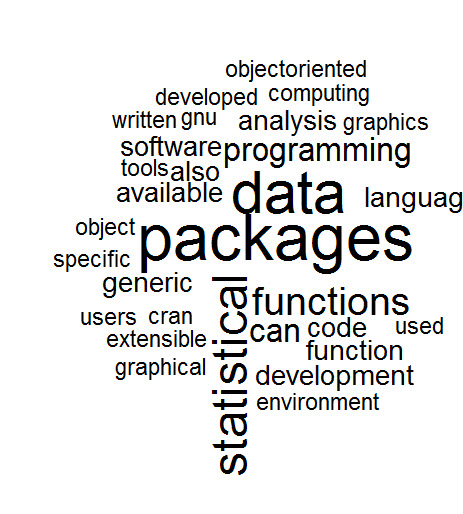To add a little more rotation to your word cloud use rot.per

```wordcloud(words = wc, scale=c(4,0.5), max.words=50,random.order=FALSE,
rot.per=0.25)```

Finally, lets add some color. We are going to use brewer.pal.  The syntax is brewer.pal(number of colors, color mix)

```cp <- brewer.pal(7,"YlOrRd")
wordcloud(words = wc, scale=c(4,0.5), max.words=50,random.order=FALSE,
rot.per=0.25, colors=cp)```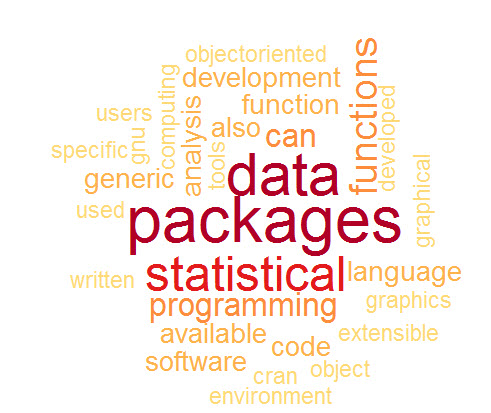# R: K-Means Clustering- Deciding how many clusters

In a previous lesson I showed you how to do a K-means cluster in R. You can visit that lesson here: R: K-Means Clustering.

Now in that lesson I choose 3 clusters. I did that because I was the one who made up the data, so I knew 3 clusters would work well. In the real world it doesn’t work that way. Choosing the right number of clusters is one of the trickier parts of performing a k-means cluster.

If you go over to Michael Grogan’s site, you will see he has a great method for figuring out how many clusters to choose. http://www.michaeljgrogan.com/k-means-clustering-example-stock-returns-dividends/

````wss <- (nrow(sample_stocks)-1)*sum(apply(sample_stocks,2,var))`
`for (i in 2:20) wss[i] <- sum(kmeans(sample_stocks,`
`centers=i)\$withinss)`
`plot(1:20, wss, type="b", xlab="Number of Clusters",`
`ylab="Within groups sum of squares")````

If you understand the code above, then great. That is a great solution for choosing the number of clusters. If, however, you are not 100% sure what is going on above, keep reading. I’ll walk you through it.

## K-Means Clustering

We need to start by getting a better understanding of what k-means clustering means. Consider this simplified explanation of clustering.

The way is works is each of the rows our data are placed into a vector.These vectors are then plotted out in space. Centroids (the yellow stars in the picture below) are chosen at random. The plotted vectors are then placed into clusters based on which centroid they are closest to.So how do you measure how good your clusters fit. (Do you need more clusters? Less clusters)? One popular metrics is the Within cluster sum of squares. R provides this as kmeans\$withinss. What this means is the distance the vectors in each cluster are from their respected centroid.

The goal is to get the is to get this number as small as possible. One approach to handling this is to run your kmeans clustering multiple times, raising the number of the clusters each time. Then you compare the withinss each time, stopping when the rate of improvement drops off. The goal is to find a low withinss while still keeping the number of clusters low.This is, in effect, what Michael Grogan has done above.

## Break down the code

Okay, now lets break down Mr. Grogan’s code and see what he is doing.

````wss <- (nrow(sample_stocks)-1)*sum(apply(sample_stocks,2,var))`
`for (i in 2:20) wss[i] <- sum(kmeans(sample_stocks,`
`centers=i)\$withinss)`
`plot(1:20, wss, type="b", xlab="Number of Clusters",`
`ylab="Within groups sum of squares")````

The first line of code is a little tricky. Let’s break it down.

`wss <- (nrow(sample_stocks)-1)*sum(apply(sample_stocks,2,var))`

sample_stocks – the data set

wss <-  – This simply assigns a value to a variable called wss

(nrow(sample_stocks)-1)  – the number of rows (nrow) in sample_stocks – 1. So if there are 100 rows in the data set, then this will return 99

sum(apply(sample_stocks,2,var)) – let’s break this down deeper and focus on the apply() function. apply() is kind of like a list comprehension in Python. Here is how the syntax works.

apply(data, (1=rows, 2=columns), function you are passing the data through)

So, let’s create a small array and play with this function. It makes more sense when you see it in action.

``` tt <- array(1:20, dim=c(10,2)) # create array with data 1 -20,
#10 rows, 2 columns
> tt
[,1] [,2]
[1,] 1 11
[2,] 2 12
[3,] 3 13
[4,] 4 14
[5,] 5 15
[6,] 6 16
[7,] 7 17
[8,] 8 18
[9,] 9 19
[10,] 10 20```

Now lets try running this through apply.

```> apply(tt, 2, mean)
 5.5 15.5```

Apply took the mean of each column. Had I used 1 as the second argument, it would have taken the mean of each row.

```> apply(tt, 1, mean)
 6 7 8 9 10 11 12 13 14 15```

Also, keep in mine, you can create your own functions to be used in apply

```apply(tt,2, function(x) x+5)
[,1] [,2]
[1,] 6 16
[2,] 7 17
[3,] 8 18
[4,] 9 19
[5,] 10 20
[6,] 11 21
[7,] 12 22
[8,] 13 23
[9,] 14 24
[10,] 15 25
```

So, what is Mr. Grogan’s doing with his apply function? apply(sample_stocks,2,var) – He is taking the variance of each column his data set.

``` apply(tt,2,var)
 9.166667 9.166667```

And by summing it: sum(apply(sample_stocks,2,var)) – he is simply adding the two values together.

``` sum(apply(tt,2,var))
 18.33333```

So, the entire first line of code using our data is:

wss <- (nrow(tt)-1)*sum(apply(tt,2,var))

wss <- (10-1) * (18.333)

```wss <- (nrow(tt)-1)*sum(apply(tt,2,var))
> wss
 165```

What this number is effectively is the within sum of squares for a data set that has only one cluster

### Next section of code

Next we will tackle the next two lines of code.

````for (i in 2:20) wss[i] <- sum(kmeans(sample_stocks,`
`centers=i)\$withinss)````

The first part is a for loop and should be simple enough. Note he doesn’t use {} to denote the inside of his loop. You can do this when your for loop is a single line, but I am going to use the {}’s anyway, as I think it makes the code a bit neater.

for (i in 2:20)  — a for loop iterating from 2 -20

for (i in 2:20) {

wss[i] <- }  – we are going to assign more values to the vector wss starting at 2 and working our way down to 20.

Remember, a single value variable in R is actually a single value vector.

```c <- 5
> c
 5
> c <- 7
> c
 5 7```

Okay, so now to the trickier code. sum(kmeans(sample_stocks, centers = i)\$withinss)

What he is doing is running a kmeans cluster for the data one time each for each value of centers (number of centroids we want) from 2 to 20 and reading the \$withinss from each run. Finally it sums all the withinss up (you will have 1 withinss for every cluster you create – number of centers)

### Plot the results

The last part of the code is plotting the results

````plot(1:20, wss, type="b", xlab="Number of Clusters",`
`ylab="Within groups sum of squares")````

plot (x, y, type= type of graph, xlab = label for x axis, ylab= label for y axis

## Let’s try it with our data

``` myData <- read.csv('cluster.csv')
StudentId TestA TestB
1 2355645.1 134 24
2 8718152.6 155 32
3 8303333.6 130 25
4 6352972.5 185 86
5 3381543.2 153 95
6 817332.4 153 81
> myData <- myData [,2:3] # get rid of StudentId column
TestA TestB
1 134 24
2 155 32
3 130 25
4 185 86
5 153 95
6 153 81```

Now lets feed this through Mr. Grogan’s code

````wss <- (nrow(myData)-1)*sum(apply(myData,2,var))`
```for (i in 2:20) {
wss[i] <- sum(kmeans(myData,```
```          centers=i)\$withinss)
```         }
`plot(1:20, wss, type="b", xlab="Number of Clusters",`
`ylab="Within groups sum of squares")````

Here is our output ( a scree plot for you math junkies out there)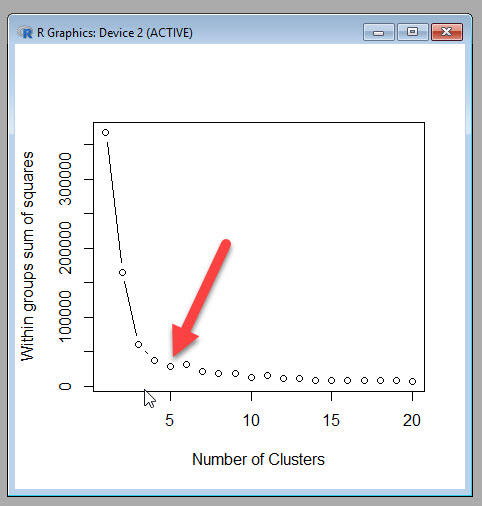Now Mr. Grogan’s plot a nice dramatic drop off, which is unfortunately not how most real world data I have seen works. I am going to chose 5 as my cut off point, because while the withinss does continue to decrease, it doesn’t seem to do so at a rate great enough to accept the added complexity of more clusters.

If you want to see how 5 clusters looks next to the three I had originally created, you can run the following code.

```myCluster <- kmeans(myData,5, nstart = 20)
myData\$cluster <- as.factor(myCluster\$cluster)
ggplot(myData, aes(TestA, TestB, color = cluster))
+ geom_point()```

5 Clusters3 Clusters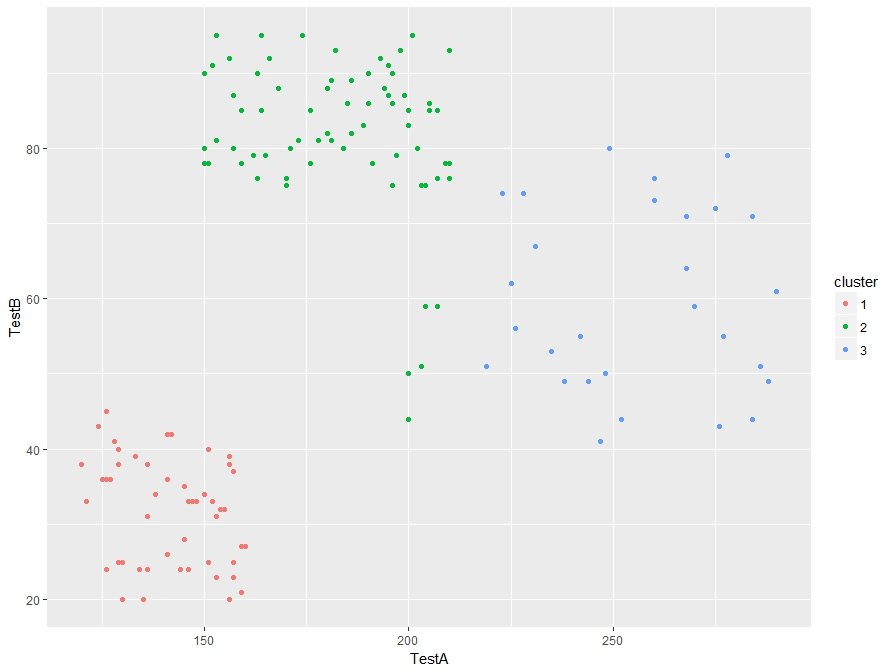I see some improvement in the 5 cluster model. So Michael Grogan’s trick for finding the number of clusters works.

# R: K-Means Clustering

Note: This is an introductory lesson with a made up data set. After you are finished with this tutorial, if you want to see a nice real world example, head on over to Michael Grogan’s website:

http://www.michaeljgrogan.com/k-means-clustering-example-stock-returns-dividends/

K Means Cluster will be our introduction to Unsupervised Machine Learning. What is Unsupervised Machine Learning exactly? Well, the simplest explanation I can offer is that unlike supervised where our data set contains a result, unsupervised does not.

Think of a simple regression where I have the square footage and selling prices (result) of 100 houses. Taking that data, I can easily create a prediction model that will predict the selling price of a house based off of square footage. – This is supervised machine learning

Now, take a data set containing 100 houses with the following data: square footage, house style, garage/no garage, but no selling price. We can’t create a prediction model since we have no knowledge of prices, but we can group the houses together based on commonalities. These groupings (clusters) can be used to gain knowledge of your data set.

I think seeing it in action will help.

Here is the data set: cluster

The data we will be looking at test results for 149 students.The task at hand is to group the students into 3 groups based on the test results. Now one thing any teacher will let you know is that some kids perform well in one subject and perhaps not so well in another. So we can’t simply group them on the score performance on one test. And when you are dealing with real world data, you might be looking at 20 -100 test/quiz scores per student.

So what we are going to do is let the computer decide how to group (or cluster) them.

To do so, we are going to be using K-means clustering. K-means clustering works by choosing random points (centroids). It then groups the data points around the centroids based which centroid the points are closest to.

## Let’s get started

```st <- read.csv(file.choose())

our dataNow let’s run the data through a Kmeans() algorithm

First, we are only going to want to focus on columns 2 and 3 in the data set since column 1 (studentID) is basically a label and provides no value in prediction.

To do this, we subset the data: st[,2:3] – which means I want all row ([,) and columns 2-3 (2:3])Now the code to make our clusters

```stCl <- kmeans(st[, 2:3], 3, nstart = 20)
stCl```

The syntax is kmeans(DATA, Number of clusters, Numbers of random starts)

Number of clusters I picked as 3 because I know this works well with the data, picking the right number usually takes a little trial and error in real life

Number of random starts is how many times you want the algorithm to be rerun (choosing new centroids each time) and choosing the result where the clusters are tightest.

Below is the output of our Kmeans – note the cluster means, this tells us the mean score for TestA and TestB set in each cluster.Hey, if you are a math junkie, this may be all you want. But if you are looking for some more practical value here, lets move on.

First, we need to add a column to our data set that shows our columns.

Now since we read our data from a csv, it is a data frame. If you can’t remember that, you can always run the command is.data.frame(st) to test it out.

Do you remember how to add a column to a data frame?

Well, there are multiple ways, but this is, in my opinion, the easiest way.

st\$cluster <- stCl\$cluster

```is.data.frame(st)
st\$cluster <- stCl\$cluster

Here is the resultNow with the clusters, you can group your students based their assigned cluster.

Technically we are done here. We have successfully grouped the students. But what if you want to make sure you did a good job. One quick check is to graph your work.

Before we can graph, we have to make sure our st\$cluster column is set as a factor, then using ggplot, we can graph it. (if you don’t have ggplot2 installed, you will need to run this line: install.packages(“ggplot2”)

```library(ggplot2)
st\$cluster <- as.factor(st\$cluster)
ggplot(st, aes(TestA, TestB, color = cluster)) + geom_point()```

And here is our output. The groups look pretty good.# R: Working with lists

Lists in R allow you to store data of different types into a single data structure. While they are extremely useful, they can be a bit confusing to work with at first.

Let’s start by creating a list. The syntax is simple enough, just add list() around the elements you want to put in your list.

```l1 <- list(24, c(12,15,19), "Dogs")
l1```

Here is the output. Note we have 3 different groupings. 1 number, 1 vector (with 3 elements) and 1 characterYou can call on each element using the element names (found in the double brackets [[]])

```l1[]
l1[]```

Here are the results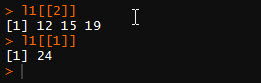Now, what if you want to call 1 element from the vector in []

You do this by adding [] to the end of the line

`l1[]`

This will give you the 3rd number in the vector found in []To make it easier to work with lists, you can rename the elements.

```names(l1)  # shows NULL since the elements have no names
names(l1) = c("Number", "Vector", "Char")
names(l1) # now shows assigned names```

Now you can call on the list using the names.

```l1\$Char # will return "Dogs"

l1\$Vector #will return the second number in the vector in the list```

```rm(l1) #deletes list
l1 <- list(Number=24,Vector = c(12,15,19), Char="Dogs")```

You can add a new element to you list via number

`l1[] = "New Element"`

Even better, you can add via new name

`l1\$Char2 <- "Cat"`

Now let’s look at our listNow we can use the names() function to give element 4 a name, or we can just get rid of it.

To delete an elements, use NULL

`l1[] <- NULL`

Now the last thing we will cover is how to subset a list

`l1[1:3] # gives us elements 1 -3`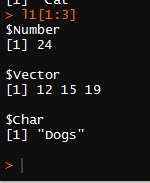We want to pick some elements out of order

```l1[c(2,4)]
l1[c("Number","Char")]```# R: Converting Factors to Numbers

R, like all programming languages, has its quirks. One of the more frustrating ones is the way it acts when trying to convert a factor into a numeric variable.

```chars <- c("12","13","14","12","11","13","12")
chars
typeof(chars)```

Here is the output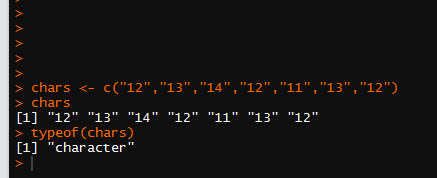Now, let’s convert this vector to a numeric vector using the function as.numeric()

```nums <- as.numeric(chars)
nums
typeof(nums)```

And here is the output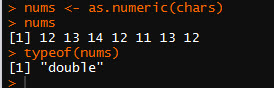As you can see it works fine.

But now let’s try it with a factor

```fac <- factor(c("12","13","14","12","11","13","12"))
fac
typeof(fac)```

Here is the output.Now look what happens when I try the as.numeric() function

```nums <- as.numeric(fac)
nums
typeof(nums)```

Check out the results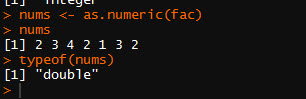While is says the type is a double, clearly the numbers are not correct.

## How to fix it?

Well, the secret is that first you need to convert the factor into a character, then into a numeric.

```nums <- as.numeric(as.character(fac))
nums
typeof(nums)```

Now check out the results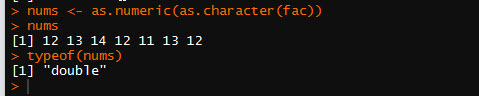Now we have the correct numbers. Just keep this little trick in mind. It has caused me some undue frustration in past.